LitePanels - An art framework - Page 9 - WoWInterface
01-21-10, 11:24 AM   #161
Katae
A Cobalt Mageweaver
Join Date: Jun 2007
Posts: 208
 Originally Posted by shinchih2001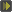i copy afker and minimap layout into layout.lua but can't work
Code:
`"n:Character r:Realm c:Class"`
Set your character name there, for example: "n:sinchih". Alternatively, Setting it to nil instead will load it on all characters.
Code:
`lpanels:ApplyLayout(nil, "Minimap", "Afker")`
Good day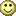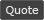01-21-10, 02:04 PM   #162
shinchih2001
A Defias Bandit
Join Date: Nov 2008
Posts: 3
 Originally Posted by KataeCode: `"n:Character r:Realm c:Class"` Set your character name there, for example: "n:sinchih". Alternatively, Setting it to nil instead will load it on all characters. Code: `lpanels:ApplyLayout(nil, "Minimap", "Afker")` Good daythanks a lot
afk is work now, but the difault minimap(round) not gone=.=01-21-10, 02:13 PM   #163
Katae
A Cobalt Mageweaver
Join Date: Jun 2007
Posts: 208
 Originally Posted by shinchih2001thanks a lot afk is work now, but the difault minimap(round) not gone=.=
You need a minimap addon for that.01-21-10, 03:26 PM   #164
shinchih2001
A Defias Bandit
Join Date: Nov 2008
Posts: 3
 Originally Posted by KataeYou need a minimap addon for that.
haha...i'm fool...=.=01-21-10, 06:44 PM   #165
brkndoll
A Kobold Labourer
Join Date: Nov 2008
Posts: 1
Thank you!!

Just wanted to say a big 'Thank you!!' for this addon.It's been fun learning to code for it.

Oh.. and here is a SS. It's a bit generic, but I love it!01-24-10, 08:45 PM #166 Shestak A Deviate Faerie Dragon Join Date: Dec 2008 Posts: 11 Hi. I can not understand. I created a panel for the buttons. Create a button that launches the setup DBM. And like that would bar was mousover. All is well, everything works, and the panel and the button. DBM run. But mousover works only on an area of the panel, where there is no text. And if you move the cursor to an area where there is text, the panel does not appear. Where can I go wrong in the code? Code: ```{ name = "Buttons panel", anchor_to = "BOTTOM", x_off = 285, y_off = 6, width = 200, height = 7, bg_alpha = 1, OnLoad = function(self) self:SetAlpha(0) end, OnEnter = function(self) self:SetAlpha(1) end, OnLeave = function(self) self:SetAlpha(0) end, }, { name = "DBM Button", parent = "Buttons panel", anchor_to = "LEFT", x_off = 0, y_off = 1, OnClick = function(self, button) if button == "LeftButton" then DBM:LoadGUI() end end, text = { { string = "DBM", anchor_to = "CENTER", outline=1, font = main_font, size = main_font_size, color = "CLASS" }, }, },```01-24-10, 08:58 PM   #167
Katae
A Cobalt Mageweaver
Join Date: Jun 2007
Posts: 208
 Originally Posted by ShestakAll is well, everything works, and the panel and the button. DBM run. But mousover works only on an area of the panel, where there is no text. And if you move the cursor to an area where there is text, the panel does not appear.
This is happening because the cursor is being intercepted by the DBM button, so the parent "thinks" that the cursor is leaving it, thus triggering the OnLeave function.

You can get around this pretty easily by checking if the cursor is not hovering over a child panel:
Code:
```OnLeave = function(self)
if GetMouseFocus():GetParent() ~= self then self:SetAlpha(0) end
end,```

Last edited by Katae : 01-24-10 at 09:10 PM.01-24-10, 09:34 PM   #168
Shestak
A Deviate Faerie Dragon
Join Date: Dec 2008
Posts: 11
 Originally Posted by KataeThis is happening because the cursor is being intercepted by the DBM button, so the parent "thinks" that the cursor is leaving it, thus triggering the OnLeave function. You can get around this pretty easily by checking if the cursor is not hovering over a child panel: Code: ```OnLeave = function(self) if GetMouseFocus():GetParent() ~= self then self:SetAlpha(0) end end,```

Now, sometimes diverting the cursor on the panel, it does not disappear.

Code:
Code:
```{	name = "Buttons panel",
anchor_to = "BOTTOM", x_off = 285, y_off = 6,
width = 200, height = 7,
bg_alpha = 1,
OnEnter = function(self) self:SetAlpha(1) end,
OnLeave = function(self)
if GetMouseFocus():GetParent() ~= self then self:SetAlpha(0) end
end,
},
{	name = "DBM Button", parent = "Buttons panel",
anchor_to = "LEFT", x_off = 0, y_off = 1,
OnClick = function(self, button)
if button == "LeftButton" then
end
end,
text = {
{ string = "DBM",
anchor_to = "CENTER",
outline=1,
font = main_font,
size = main_font_size,
color = "CLASS"
},
},
},```01-28-10, 04:56 PM   #169
Tandurin
A Fallenroot Satyr
Join Date: Apr 2009
Posts: 25
Hehe sry to bust in again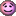I wrote a new code after my hard-drive crashed. Though again it doesn't show up =S.

I thought I did everything right this time...

here's the code

Code:
```lpanels:CreateLayout("Chat", {
-- Main Chat Background
{	name = "Chat",
strata = "BACKGROUND", level = 0,
x_off = 0, y_off = "1%",
width = "20%", height = 125,
bg_color = "1 1 1", bg_alpha = 0.5,
anchor_to = "BOTTOMLEFT",
border_size = 1, Border_color = "1 1 1",
},
}),

lpanels:CreateLayout("Combat", {
-- Main Chat Background
{	name = "Combat",
strata = "BACKGROUND", level = 0,
x_off = 0, y_off = "-1%",
width = "20%", height = 125,
bg_color = "1 1 1", bg_alpha = 0.5,
anchor_to = "BOTTOMRIGHT",
border_size = 1, Border_color = "1 1 1",
},
}),

lpanels:CreateLayout("Hotbar", {
-- Main Chat Background
{	name = "Hotbar",
strata = "BACKGROUND", level = 0,
x_off = 0, y_off = "1%",
width = "55%", height = 55,
bg_color = "1 1 1", bg_alpha = 0.5,
anchor_to = "BOTTOM",
border_size = 1, Border_color = "1 1 1",
},
}), lpanels:ApplyLayout(nil, "Chat", "Combat", "Hotbar");```01-28-10, 05:08 PM #170 Katae A Cobalt Mageweaver Join Date: Jun 2007 Posts: 208 These commas, delete them: }),02-03-10, 05:13 PM   #171
crashbeaga
A Kobold Labourer
Join Date: Oct 2008
Posts: 1
Guys I have a question, I have my UI set up and i placed a bottom panel on the (of course) bottom of screen which is 200px height.

and using bartender as actionbar mod, i like to use the blizzard vehicle ui as default, so what i wanted to do is, when i enter on vehicle, when that frame pops up, a black panel shows behind which hides my current panel (its texture)

i guess u can have a clear view from these picz

http://img692.imageshack.us/img692/8...0310231112.jpg

http://img442.imageshack.us/img442/6...0310231139.jpg

thanks guys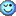02-03-10, 05:41 PM #172 Katae A Cobalt Mageweaver Join Date: Jun 2007 Posts: 208 You'll need to script a little to watch the UNIT_ENTERED_VEHICLE and UNIT_EXITED_VEHICLE events. You'll also need to set the strata of this new panel to be just high enough to cover your textures. As an example: Code: ```{ x_off = 0, y_off = 0, bg_color = "0 0 0", bg_alpha = 0, strata = "HIGH", OnLoad = function(self) self:RegisterEvent'UNIT_ENTERED_VEHICLE' self:RegisterEvent'UNIT_EXITED_VEHICLE' if UnitHasVehicleUI'player' then self.bg:SetAlpha(1) end end, OnEvent = function(self, event, unit) if unit == "player" then if event == "UNIT_ENTERED_VEHICLE" then self.bg:SetAlpha(1) else self.bg:SetAlpha(0) end end end, }``` Last edited by Katae : 02-03-10 at 05:48 PM. Reason: variables02-20-10, 11:13 AM   #173
Star1814
A Defias Bandit
Join Date: Oct 2008
Posts: 3
Border disappearing

Why, oh why, do the border keep disappearing and reappearing when the values of x_off and y_off are changed? Am trying to parent a panel with a background color and border to a Bartender4 bar (well, button to be specific as there's no frame for the bar, sucks -- any suggestion on that anyone?), and it's driving me insane.03-07-10, 06:44 PM   #174
Breetard
A Murloc Raider
Join Date: Mar 2010
Posts: 7
 Originally Posted by KataeGradients with a stats displayI'll start with some scripts I've been using. This is the same layout code I used for the stats display, copy and paste this into the default or your character's profile. This also demonstrates the built-in class coloring. If you want to only use the gradient box, you could remove the stats panel (name = "Stats") and tweak the dimensions, locations, and colors. (Font in the screenshot is 04B_03) Code: ```lpanels:CreateLayout("Stats", { -- Stat Container { name = "Container", anchor_to = "TOP", width = 280, height = 20, x_off = 0, y_off = -30, bg_alpha = 0, }, -- Left mid-section of the box { name = "BoxL", parent = "Container", anchor_to = "LEFT", width = "50%", height = "100%", gradient = "H", bg_color = "CLASS", gradient_color = "CLASS", bg_alpha = 0, gradient_alpha = 0.2, }, -- Right mid-section { name = "BoxR", parent = "Container", anchor_to = "RIGHT", width = "50%", height = "100%", gradient = "H", bg_color = "CLASS", gradient_color = "CLASS", bg_alpha = 0.2, gradient_alpha = 0, }, -- Top Left gradient line { name = "LineTL", parent = "BoxL", anchor_to = "TOP", width = "100%", height = 1, gradient = "H", bg_color = "CLASS", gradient_color = "CLASS", bg_alpha = 0, gradient_alpha = 0.8, }, -- Top right line { name = "LineTR", parent = "BoxR", anchor_to = "TOP", width = "100%", height = 1, gradient = "H", bg_color = "CLASS", gradient_color = "CLASS", bg_alpha = 0.8, gradient_alpha = 0, }, -- Bottom left line { name = "LineBL", parent = "BoxL", anchor_to = "BOTTOM", width = "100%", height = 1, gradient = "H", bg_color = "CLASS", gradient_color = "CLASS", bg_alpha = 0, gradient_alpha = 0.8, }, -- Bottom right line { name = "LineBR", parent = "BoxR", anchor_to = "BOTTOM", width = "100%", height = 1, gradient = "H", bg_color = "CLASS", gradient_color = "CLASS", bg_alpha = 0.8, gradient_alpha = 0, }, -- Text display { name = "Stats", parent = "Container", anchor_to = "CENTER", anchor_from = "CENTER", text = { string = function() -- monies local gold = format("%.1f|cffffd700g|r",GetMoney()/10000) -- durability local durability = 100 for i = 1, 11 do if GetInventoryItemDurability(i) ~= nil then local dur, max = GetInventoryItemDurability(i) local perc = dur / max * 100 if perc < durability then durability = floor(perc) end end end -- fps local fps = floor(GetFramerate()) -- memory local memory = 0 UpdateAddOnMemoryUsage() for i = 1, GetNumAddOns() do if IsAddOnLoaded(i) then memory = memory + GetAddOnMemoryUsage(i) end end memory = format("%.1f", memory/1024) -- latency local latency = select(3,GetNetStats()) return format(":: %s :: %s%%dur :: %sfps :: %smB :: %sms ::", gold, durability, fps, memory, latency) end, update = 1, size=10, shadow=1, font = "Fonts\\FRIZQT__.TTF", } } }); lpanels:ApplyLayout(nil, "Stats")```
Say if I wanted to add the server time into that, how would I go about doing that (so tottaly noob to this)

btw love this! makes my ui look so clean!03-07-10, 06:59 PM   #175
xandora
A Chromatic Dragonspawn
Join Date: Feb 2009
Posts: 188
Try using GetGameTime() somewhere.

http://www.wowwiki.com/API_GetGameTime
__________________Last edited by xandora : 03-07-10 at 07:03 PM. Reason: Extended info.03-07-10, 09:08 PM   #176
Breetard
A Murloc Raider
Join Date: Mar 2010
Posts: 7
 Originally Posted by xandoraTry using GetGameTime() somewhere. http://www.wowwiki.com/API_GetGameTime
Didnt seem to work, do you think you could help me by adding it in the code?03-07-10, 09:41 PM   #177
Katae
A Cobalt Mageweaver
Join Date: Jun 2007
Posts: 208
 Originally Posted by BreetardDidnt seem to work, do you think you could help me by adding it in the code?
You can just add this code, then add the variables hour and minute to the end of the existing format function, like so:

Code:
```-- Server Clock
local hour, minute, aptime, pm = GetGameTime(), true
if aptime then
if hour == 0 then hour = 12 end
if hour > 12 then hour = hour - 12 end
if hour >= 12 then pm = true end
end

return format(":: %s :: %s%%dur :: %sfps :: %smB :: %sms :: %s:%s%s ::", gold, durability, fps, memory, latency, hour, minute, aptime and pm and "pm" or "am" or "")```
Should work, did not test.03-07-10, 11:11 PM   #178
Breetard
A Murloc Raider
Join Date: Mar 2010
Posts: 7
 Originally Posted by KataeYou can just add this code, then add the variables hour and minute to the end of the existing format function, like so: Code: ```-- Server Clock local hour, minute, aptime, pm = GetGameTime(), true if aptime then if hour == 0 then hour = 12 end if hour > 12 then hour = hour - 12 end if hour >= 12 then pm = true end end return format(":: %s :: %s%%dur :: %sfps :: %smB :: %sms :: %s:%s%s ::", gold, durability, fps, memory, latency, hour, minute, aptime and pm and "pm" or "am" or "")``` Should work, did not test.
Its all gone now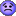this is what I have.

Code:
```lpanels:CreateLayout("Stats", {
-- Stat Container
{    name = "Container",
anchor_to = "BOTTOM",
width = 1020, height = 20,
x_off = 0, y_off = 0,
bg_alpha = 0,
},
-- Left mid-section of the box
{    name = "BoxL", parent = "Container",
anchor_to = "LEFT",
width = "50%", height = "100%",
bg_color = "CLASS", gradient_color = "CLASS",
bg_alpha = 0, gradient_alpha = 0.2,
},
-- Right mid-section
{    name = "BoxR", parent = "Container",
anchor_to = "RIGHT",
width = "50%", height = "100%",
bg_color = "CLASS", gradient_color = "CLASS",
bg_alpha = 0.2, gradient_alpha = 0,
},
{    name = "LineTL",
parent = "BoxL", anchor_to = "TOP",
width = "100%", height = 1,
bg_color = "CLASS", gradient_color = "CLASS",
bg_alpha = 0, gradient_alpha = 0.8,
},
-- Top right line
{    name = "LineTR",
parent = "BoxR", anchor_to = "TOP",
width = "100%", height = 1,
bg_color = "CLASS", gradient_color = "CLASS",
bg_alpha = 0.8, gradient_alpha = 0,
},
-- Bottom left line
{    name = "LineBL",
parent = "BoxL", anchor_to = "BOTTOM",
width = "100%", height = 1,
bg_color = "CLASS", gradient_color = "CLASS",
bg_alpha = 0, gradient_alpha = 0.8,
},
-- Bottom right line
{    name = "LineBR",
parent = "BoxR", anchor_to = "BOTTOM",
width = "100%", height = 1,
bg_color = "CLASS", gradient_color = "CLASS",
bg_alpha = 0.8, gradient_alpha = 0,
},
-- Text display
{    name = "Stats", parent = "Container",
anchor_to = "CENTER", anchor_from = "CENTER",
text = {
string = function()
-- monies
local gold = format("%.1f|cffffd700g|r",GetMoney()/10000)

-- durability
local durability = 100
for i = 1, 11 do
if GetInventoryItemDurability(i) ~= nil then
local dur, max = GetInventoryItemDurability(i)
local perc = dur / max * 100
if perc < durability then
durability = floor(perc)
end
end
end

-- fps
local fps = floor(GetFramerate())

-- memory
local memory = 0
for i = 1, GetNumAddOns() do
end
end
memory = format("%.1f", memory/1024)

-- latency
local latency = select(3,GetNetStats())

-- Server Clock
local hour, minute, aptime, pm = GetGameTime(), true
if aptime then
if hour == 0 then hour = 12 end
if hour > 12 then hour = hour - 12 end
if hour >= 12 then pm = true end
end

return format(":: %s :: %s%%dur :: %sfps :: %smB :: %sms :: %s:%s%s ::", gold, durability, fps, memory, latency, hour, minute, aptime and pm and "pm" or

"am" or "")
end, update = 1,
}
}
}); lpanels:ApplyLayout(nil, "Stats")```03-07-10, 11:25 PM #179 Katae A Cobalt Mageweaver Join Date: Jun 2007 Posts: 208 Code: `"am" or "")` Should be on the same line with format. Code: `return format(":: %s :: %s%%dur :: %sfps :: %smB :: %sms :: %s:%s%s ::", gold, durability, fps, memory, latency, hour, minute, aptime and (pm and "pm" or "am") or "")` Last edited by Katae : 03-07-10 at 11:28 PM.03-08-10, 03:30 AM   #180
Breetard
A Murloc Raider
Join Date: Mar 2010
Posts: 7
 Originally Posted by KataeCode: `"am" or "")` Should be on the same line with format. Code: `return format(":: %s :: %s%%dur :: %sfps :: %smB :: %sms :: %s:%s%s ::", gold, durability, fps, memory, latency, hour, minute, aptime and (pm and "pm" or "am") or "")`
ffs I must be super noob because I'm not getting it at all. I'm sorry >.<

Code:
```lpanels:CreateLayout("Stats", {
-- Stat Container
{    name = "Container",
anchor_to = "BOTTOM",
width = 1020, height = 20,
x_off = 0, y_off = 0,
bg_alpha = 0,
},
-- Left mid-section of the box
{    name = "BoxL", parent = "Container",
anchor_to = "LEFT",
width = "50%", height = "100%",
bg_color = "CLASS", gradient_color = "CLASS",
bg_alpha = 0, gradient_alpha = 0.2,
},
-- Right mid-section
{    name = "BoxR", parent = "Container",
anchor_to = "RIGHT",
width = "50%", height = "100%",
bg_color = "CLASS", gradient_color = "CLASS",
bg_alpha = 0.2, gradient_alpha = 0,
},
{    name = "LineTL",
parent = "BoxL", anchor_to = "TOP",
width = "100%", height = 1,
bg_color = "CLASS", gradient_color = "CLASS",
bg_alpha = 0, gradient_alpha = 0.8,
},
-- Top right line
{    name = "LineTR",
parent = "BoxR", anchor_to = "TOP",
width = "100%", height = 1,
bg_color = "CLASS", gradient_color = "CLASS",
bg_alpha = 0.8, gradient_alpha = 0,
},
-- Bottom left line
{    name = "LineBL",
parent = "BoxL", anchor_to = "BOTTOM",
width = "100%", height = 1,
bg_color = "CLASS", gradient_color = "CLASS",
bg_alpha = 0, gradient_alpha = 0.8,
},
-- Bottom right line
{    name = "LineBR",
parent = "BoxR", anchor_to = "BOTTOM",
width = "100%", height = 1,
bg_color = "CLASS", gradient_color = "CLASS",
bg_alpha = 0.8, gradient_alpha = 0,
},
-- Text display
{    name = "Stats", parent = "Container",
anchor_to = "CENTER", anchor_from = "CENTER",
text = {
string = function()
-- monies
local gold = format("%.1f|cffffd700g|r",GetMoney()/10000)

-- durability
local durability = 100
for i = 1, 11 do
if GetInventoryItemDurability(i) ~= nil then
local dur, max = GetInventoryItemDurability(i)
local perc = dur / max * 100
if perc < durability then
durability = floor(perc)
end
end
end

-- fps
local fps = floor(GetFramerate())

-- memory
local memory = 0
for i = 1, GetNumAddOns() do
end
end
memory = format("%.1f", memory/1024)

-- latency
local latency = select(3,GetNetStats())

-- Server Clock
local hour, minute, aptime, pm = GetGameTime(), true
if aptime then
if hour == 0 then hour = 12 end
if hour > 12 then hour = hour - 12 end
if hour >= 12 then pm = true end
end

return format(":: %s :: %s%%dur :: %sfps :: %smB :: %sms :: %s:%s%s ::",  gold, durability, fps, memory, latency, hour, minute, aptime and (pm and  "pm" or "am") or "")
end, update = 1,
It just takes away the font and there is a blank barThread Tools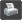Show Printable Version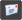Email this Page Display Modes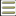Linear Mode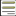Switch to Hybrid Mode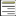Switch to Threaded Mode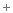Posting Rules You may not post new threads You may not post replies You may not post attachments You may not edit your posts vB code is On Smilies are On [IMG] code is On HTML code is Off